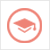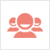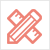﻿ 更多范文-cs代写：Statistic Simulation-51Due留学教育

#### 服务承诺资金托管原创保证实力保障24小时客服使命必达

#### 关于我们

51Due提供Essay，Paper，Report，Assignment等学科作业的代写与辅导，同时涵盖Personal Statement，转学申请等留学文书代写。51Due将让你达成学业目标51Due将让你达成学业目标51Due将让你达成学业目标51Due将让你达成学业目标

#### 名企实习积累工作经验多元化文化交流专业实操技能建立人际资源圈

# cs代写：Statistic Simulation

2018-03-14 来源: 51due教员组 类别: 更多范文Write a C++ program to implement the following simulation.

Consider a square board with N rows of N bins. S stones ( 0 < S < N*N ) are placed randomly into the bins on the board, only one stone may be placed in any particular bin. The probability that a bin will contain a stone after all the stones have been placed on the board is the same for each bin and is PS = S / ( N * N ).

You will analyze the randomness of the distribution of stones on the board. The measure of randomness you will use is not a particularly good measure but a simple one to compute. The measure of randomness involves counting the number of neighboring bins that contain stones for each bin on the board. The expected values for the probability of each number of neighbors can be easily calculated based on statistics as explained in the description of the CalcDistribution function described below. The measure of randomness is also simple to calculate for an actual array containing stones as explained in the description of the function CountDistribution.

First you will need to generate the matrix representing the stones on the board. A dynamically allocated 2-D matrix of integers is used. Each of the bins on the board is represented by one of the elements in the array. A 1 in the array element represents a stone in the bin. A 0 in the array element represents an empty bin. You will generate two different dynamically allocated matrices. The first matrix will contain a “random” set of stones. The process for generating a “random” distribution of stones is explained below in the description of the function ArrayRandFill. The second matrix will contain a specific non-random pattern explained below in the description of the function ArrayP1Fill.

You will then measure the probability distribution for each of your two grids. You will also calculate the expected distribution.

The grids holding the stones and the probability distributions will be printed to the screen.

Finally, you will run the experiment with the random grid many times, averaging the results to get a more realistic estimate of the observed random statistics.

Your program will include a main function and each of the functions with the prototypes given below. The prototypes given may not be changed.

int MyFactorial( int numb );

double Permutation( int totNumObj, int numObjChosen );

void ArrayP1Fill( int sizeGrid, int** randGrid);

int ArrayRandFill( int sizeGrid, int numStones, int** randGrid, unsigned int seed);

void PrintSqArray( int sizeGrid, int elementWidth, int** myGrid);

void PrintArray(double myDist[][MAX_INTERIOR_NEIGH + 1])

void CountDistribution( int sizeGrid, int** randGrid, double distMeas[][MAX_INTERIOR_NEIGH + 1] )

void CalcDistribution( int sizeGrid, int numStones, double distCalc[][MAX_INTERIOR_NEIGH + 1] );

Error handling in all functions should be done using exceptions. Any error in a function should throw an exception (a string containing the error message). All exceptions should be caught and handled in the main program. All error messages generated by any function should be printed in the main function. No function should terminate the program.

A full call stack should be generated. To generate the call stack follow these steps:

When an error occurs, the function in which the error occurs should throw an exception containing the error message.

Each function (except main) should catch all exceptions containing error messages generated by functions it calls. Each captured exception should print the message “Called from functionName” and then rethrow the captured exception. The word functionName in the message “Called from functionName” should be replaced with the name of the function printing the message.

The main function should catch all exceptions containing error messages generated by functions it calls. Main should then print the original error message thrown by the function that failed

MAIN

Your main function should do each of the following:

Use the following global constants

const int MAX_SIZEGRID = 50; // size of largest board allowed

const int MAX_INTERIOR_NEIGH = 8; // number of neighbor bins for each interior bin

const int MAX_EDGE_NEIGH = 5; // number of neighbor bins for each edge bin

const int MAX_CORNER_NEIGH = 3; // number of neighbor bins for each corner bin

const int MAX_TYPES_NEIGH = 3;

Use the following local constants

const int MAX_REPEATS = 100000;

const int MAX_TRIES = 4;

Declare at least two arrays to hold distributions. These arrays will be MAX_INTERIOR_NEIGH+1 columns by MAX_TYPES_NEIGH rows. Also declare pointers needed for dynamic allocation of two 2-D grid arrays (to hold stones) whose dimensions will be determined when the program runs.

Prompt for the number of bins along each edge of the board using the prompt below. Check that an integer was read and was in the range 0 < bins along each edge <= MAX_SIZEGRID. If an integer was not read or if the integer that was read was out of range print the appropriate error message from the list below then re-prompt, reread and retest the number of bins. Read the number of bins a maximum of four times. After four failed reads terminate the program.

Enter the number of bins along the edge of the square board:

ERROR: the number of bins along the edge of the square board was not an integer

ERROR: illegal number of bins along the edge of the square board.

ERROR: Too many tries reading the number of bins

Additional constants may also be needed

Prompt for the number of stones using the prompt below.Check that an integer was read and was in the range 0 < # of stones <= MAX_(bins along each edge)2. If an integer was not read or if the integer that was read was out of range print the appropriate error message from the list below then re-prompt, reread and retest the number of stones. Read the number of stones a maximum of four times. After four failed reads terminate the program.

Enter the number of stones:

ERROR: the number of stones was not an integer

ERROR: illegal number of stones.

ERROR: Too many tries reading the number of stones

Prompt for the seed of the random number generator using the prompt below.Check that an integer was read and was in the range 1 < seed <= RAND_MAX. If an unsigned integer was not read or if the unsigned integer that was read was out of range print the appropriate error message from the list below then reprompt, reread and retest the number of bins. Read the number of bins a maximum of four times. After four failed reads terminate the program.

Enter the seed for the random number generator:

ERROR: seed was not an integer

ERROR: illegal seed.

ERROR: Too many tries reading the seed.

Prompt for the number of times the random grid experiment should be repeated to determine the final statistics using the prompt below.Check that an integer was read and was in the range 0 < number of repeats <= MAX_REPEATS. If an integer was not read or if the integer that was read was out of range print the appropriate error message from the list below then reprompt, reread and retest the number of bins. Read the number of bins a maximum of four times. After four failed reads terminate the program.

Enter the number of repeats for the test:

ERROR: number of test repeats was not an integer

ERROR: illegal number of test repeats.

ERROR: Too many tries reading the number of test repeats.

Dynamically allocate two 2-D arrays to hold the grid (the bins).If any of the necessary dynamic allocations fail terminate the program and print the error message below. The allocations should be done inside a try block, and all possible failures should be handled in the corresponding catch block,. Note that if some of the arrays have been successfully allocated before the failure they must be deleted before the program is terminated.

ERROR: dynamic memory could not be allocated:

In a single try block do the following calculations and print the following results. Use the grid size, seed,number of stones and number of repeats read earlier in the program as input.

Calculate the theoretical distribution using CalcDistribution

Print the calculated distribution using PrintArray

Fill the first dynamic 2-D array with random data using ArrayRandFill

Print the contents of the first dynamic 2-D array using PrintSqArray

Measure the distribution of neighbors in the first dynamic 2-D array using CountDistribution

Print the distribution measured in step e usingPrintArray

Fill the second dynamic array with the checkerboard pattern using ArrayP1Fill

Print the contents of the second dynamic 2-D array using PrintSqArray

Measure the distribution of the data in the second dynamic 2-D array using CountDistribution

Print the distribution measured in step Iusing PrintArray

Next you will do a more accurate measurement of the distribution. To obtain a more accurate measurement you will take an average over measurements for many different random arrays. Set up a loop to repeat the following steps for each of the repeats of the test. You have already read the number of repeats that are to be performed.

Zero an array of the correct size to hold the probability distribution. This array will be referred to as the sum array.

For each repeat

Fill one of the 2-D dynamic arrays with random numbers using ArrayRandFill (remember to set the grid array elements to 0 before doing this).

Measure the distribution for this array using CountDistribution. (Remember to 0 the distribution array elements first)

iii. Add each element of the array holding the distribution to the same element of the sum array (see step l for definition of the sum array)

The value returned by ArrayRandFill will be used as the seed for the next call to ArrayRandFill

Divide each element of the sum array by the number of times the distribution was calculated in step m.

Print the sum array using PrintArray

Catch any exceptions thrown within the called functions. If any exceptions are caught

Print the error message

Terminate the program

Delete your dynamic arrays

51due留学教育原创版权郑重声明：原创assignment代写范文源自编辑创作，未经官方许可，网站谢绝转载。对于侵权行为，未经同意的情况下，51Due有权追究法律责任。主要业务有assignment代写、essay代写、paper代写、cs代写服务。

51due为留学生提供最好的assignment代写服务，亲们可以进入主页了解和获取更多assignment代写范文 提供作业代写服务，详情可以咨询我们的客服QQ：800020041。